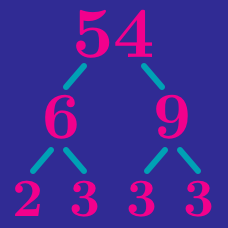Number Theory

Prime Factorization and Divisors

How many positive divisors does 20 have?

Which of these numbers has the most divisors?

Which of these numbers has the most divisors?

$\begin{array} {c|c|c} n & \text{Prime Factorization} & \text{\# of Divisors} \\ \hline 20 & 2^2 \times 5^1 & 6 \\ 30 & 2^1 \times 3^1 \times 5^1 & 8 \\ 50 & 2^1 \times 5^2 & 6 \\ 60 & 2^2 \times 3^1 \times 5^1 & 12 \\ 90 & 2^1 \times 3^2 \times 5^1 & 12 \\ 150 & 2^1 \times 3^1 \times 5^2 & 12 \\ 210 & 2^1 \times 3^1 \times 5^1 \times 7^1 & ? \end{array}$ How many divisors does 210 have?

\begin{aligned} 210 &= 2^1 \times 3^1 \times 5^1 \times 7^1\\ \\ 5 &= 5^1\\ 15 &= 3^1 \times 5^1\\ 28 &= 2^2 \times 7^1\\ 35 &= 5^1 \times 7^1\\ 42 &= 2^1 \times 3^1 \times 7^1 \end{aligned} Which is not a divisor of 210?

×